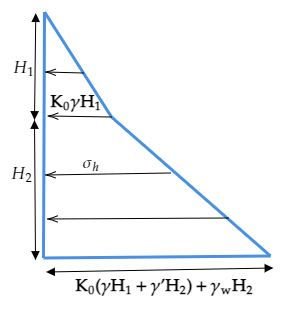top of page
Search
• APSEd

# Rankine's Earth Pressure Theory | Special Cases and Formulas

Updated: Jan 21, 2021

To analyse the pressure relation between retaining wall and soil mass, the most commonly used theory is Rankine's Earth Pressure Theory. Three conditions are analysed - Wall at rest, Active Earth Pressure and Passive Earth Pressure.

Apart from these basic conditions, there are some subcases like sloping backfill, backfill with surcharge and submerged backfill for cohesive and cohesionless soil. This is the key highlight of the article.Inclined backfill, stratified submerged backfill and backfill with surcharge

We will directly jump to the important subcases as it is an extension of this article on Rankine's earth Pressure Theory. The subcases are-

## Earth Pressure at Rest for Partially Submerged Soil

Consider H is the height of retaining wall and 'H1' is the depth(from top) below which water table is there.

At any depth Z (Z H1), the vertical pressure, σv = γZ

So, the lateral earth pressure, σh = KoγZ

At any depth Z (H Z H1), the effective vertical pressure, σv = γH1 + γ'(Z - H1)

So, the effective lateral earth pressure, σh = Ko[γH1 + γ'(Z - H1)]

Again the lateral pressure due to pore water is, u = γw(Z-H1)

Where, γ: the unit weight of soil

γ' = γsat - γw: the effective unit weight of soil

γsat: Saturated unit weight of soil

γw: Unit weight of water = 9.81 kN/m^3

Ko: At rest earth pressure constant

The variation of lateral earth pressure with respect to depth(pressure distribution diagram) for this condition can be represented as below-Pressure distribution diagram

The total maximum lateral pressure due to earth and water = Ko(γH1 + γ'H2) + γw(Z-H1)

The force per unit length(Po) of the retaining wall is horizontal and equals to the total area of pressure distribution diagram.## Cohesionless Soil Supporting a Surcharge | Rankine's Active State

Consider a frictionless retaining wall of height H, supporting cohesionless backfill. The backfill is also supporting a surcharge pressure of q unit per unit area.

At any depth Z, the vertical pressure due to surcharge = q

At any depth Z, the vertical pressure due to earth = γZ

The effective vertical pressure, σv = (q + γZ)

So, the effective lateral pressure, σh = σvKa = Ka(q + γZ)

Where, γ: the unit weight of soil

Ka: Active state lateral earth pressure constant = tan^2(45 - φ/2)

The variation of lateral earth pressure with respect to depth(pressure distribution diagram) for this condition can be represented as below-Pressure distribution diagram

The force per unit length(Po) of the retaining wall is horizontal and equals to the total area of pressure distribution diagram.

Po = 0.5Ka(q + γH)H

## Cohesionless Soil Supporting a Surcharge | Rankine's Passive State

Consider a frictionless retaining wall of height H, supporting cohesionless backfill. The backfill is also supporting a surcharge pressure of q unit per unit area.

At any depth Z, the vertical pressure due to surcharge = q

At any depth Z, the vertical pressure due to earth = γZ

The effective vertical pressure, σv = (q + γZ)

So, the effective lateral pressure, σh = σvKp = Kp(q + γZ)

Where, γ: the unit weight of soil

Kp: Passive state lateral earth pressure constant = tan^2(45 + φ/2)

The variation of lateral earth pressure with respect to depth(pressure distribution diagram) for this condition can be represented as below-Pressure distribution diagram

The force per unit length(Po) of the retaining wall is horizontal and equals to the total area of pressure distribution diagram.

Po = 0.5Kp(q + γH)H

## Sloping or Inclined Backfill

In all of the above cases, we considered the retaining wall with vertical backs and horizontal backfills. In some cases, however, the backfill may be continuously sloping at an angle α with the horizontal.

Under this condition, for active and passive pressure state, the direction of Rankine's active or passive pressures are no longer horizontal. Rather, they are inclined at an angle α with the horizontal.

In this condition everything remains, as usual, the only significant change occurs in the formula of Ka and Kp.Where, α: Angle of inclination of backfill

φ: Angle of internal friction of soil

Ka: Active earth pressure constant

Kp: Passive earth pressure constant

## Problem on Rankine's earth pressure theory

Try this problem out!

Winding up here! Let us know by commenting, what all you want next.

Subscribe to APSEd Blog and get to know the latest.